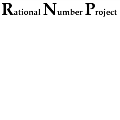This is a series of lessons from the Rational Number Project (http://www.cehd.umn.edu/ci/rationalnumberproject/default.html) including teacher notes, activities ready to be copied.

The Rational Number Project (RNP) is a cooperative research and development project funded by the National Science Foundation. Project personnel have been investigating children’s learning of fractions, ratios, decimals and proportionality since 1979. This book of fraction lessons is the product of several years of working with children in classrooms as we tried to understand how to organize instruction so students develop a deep, conceptual understanding of fractions.

You may pick and choose the lessons that you would like to try and do not need to complete them all in order.

### Rational Number Project - Initial Fraction Ideas

Created on 10/10/2014 by Scott Adamson

This is a series of lessons from the Rational Number Project (http://www.cehd.umn.edu/ci/rationalnumberproject/default.html) including teacher notes, activities ready to be copied.

The Rational Number Project (RNP) is a cooperative research and development project funded by the National Science Foundation. Project personnel have been investigating children’s learning of fractions, ratios, decimals and proportionality since 1979. This book of fraction lessons is the product of several years of working with children in classrooms as we tried to understand how to organize instruction so students develop a deep, conceptual understanding of fractions.

You may pick and choose the lessons that you would like to try and do not need to complete them all in order. Each of the following lessons and the Teacher's Guide has been uploaded here.

A brief description of the lessons:

 Teacher's Guide 22 pages 432 KB Lesson 1 Exploring with Fraction Circles 4 pages 220 KB Lesson 2 Modeling with Fraction Circles 6 pages 180 KB Lesson 3 Modeling with Fraction Circles 5 pages 164 KB Lesson 4 Comparing Paper Folding and Circles 17 pages 740 KB Lesson 5 Connecting Models with Symbols 9 pages 464 KB Lesson 6 Comparing Unit Fractions 7 pages 368 KB Lesson 7 Paper Folding and Comparing Fractions 6 pages 460 KB Lesson 8 Fraction Circles and Equivalence 9 pages 516 KB Lesson 9 Fraction Circles, Paper Folding and Equivalence 8 pages 384 KB Lesson 10 Paper Folding and Equivalence 9 pages 376 KB Lesson 11 Comparing Fractions to One-half 5 pages 368 KB Lesson 12 Introducing the Chip Model 5 pages 200 KB Lesson 13 Using the Chip Model 4 pages 232 KB Lesson 14 Using the Chip Model 5 pages 184 KB Lesson 15 Chips and Equivalence 9 pages 344 KB Lesson 16 Fraction Circles and Reconstructing the Unit 5 pages 252 KB Lesson 17 Fraction Circles and Fractions Greater than One 9 pages 356 KB Lesson 18 Fraction Circles and Names for One-half 5 pages 384 KB Lesson 19 Estimation and Fraction Addition 5 pages 256 KB Lesson 20 Fraction Circles and Fraction Addition 7 pages 320 KB Lesson 21 Estimation and Fraction Subtraction 7 pages 384 KB Lesson 22 Fraction Circles and Fraction Subtraction 4 pages 200 KB Lesson 23 Fraction Number Sense, Addition and Subtraction 4 pages 260 KB

Appendix - the complete appendix - 69 pages - 1.8 MB